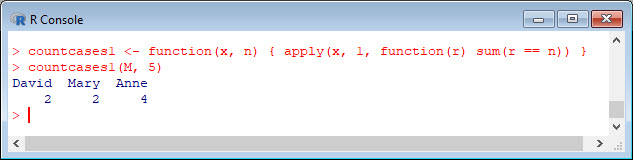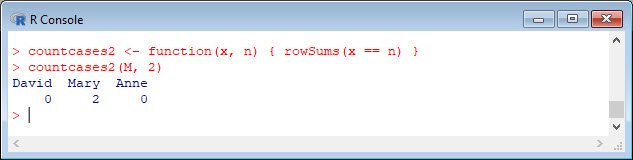SPSS has the Count Values within Cases option, but R does not have an equivalent function. Here are two functions that you might find helpful, each of which counts values within cases inside a rectangular array. For example, you might have a dataset consisting of responses to a questionnaire involving multiple Likert items scored 1 to 5. You may wish to know the number of items for which respondents selected a 5. Note the syntax for creating a function, which I will not discuss here. Copy and paste the two functions into the R workspace. They both do the same job, so that you can choose either of them for your own data analysis.
`countcases1 <- function(x, n) { apply(x, 1, function(r) sum(r == n)) }`
OR
`countcases2 <- function(x, n) { rowSums(x == n) }`
Now let’s use these functions to count elements within a rectangular array. Let’s use the following array `M`.
```M <- structure(c(1, 4, 5, 4, 5, 5, 3, 2, 5, 5, 5, 4, 5, 2, 5), .Dim = c(3L, 5L), .Dimnames = list(c("David", "Mary", "Anne"), NULL)) colnames(M) <- c("Item1", "Item2", "Item3", "Item4", "Item5") M```Let’s count the fives along the rows of `M`.
`countcases1(M, 5)`Let’s use the other function to count the twos.
`countcases2(M, 2)`Each of the two functions has produced a vector of counts. Now let’s pick out the number of fives in the first row using square brackets.
`countcases1(M, 5)`OR:
`countcases1(M, 5)["David"]`That wasn’t so hard! In Blog 20 I will present another tip for data analysis in R.
See you later!
David

#### Annex: R codes used

```# Create two functions for counting cases within an area.
countcases1 <- function(x, n) { apply(x, 1, function(r) sum(r == n)) }
countcases2 <- function(x, n) { rowSums(x == n) }

# Create a rectangular area.
M <- structure(c(1, 4, 5, 4, 5, 5, 3, 2, 5, 5, 5, 4, 5, 2, 5), .Dim = c(3L, 5L), .Dimnames = list(c("David", "Mary", "Anne"), NULL))
colnames(M) <- c("Item1", "Item2", "Item3", "Item4", "Item5")
M

# Count the fives along the rows of M.
countcases1(M, 5)

# Count the twos along the rows of M.
countcases2(M, 2)

# Pick out the number of fives in the first row.
countcases1(M, 5)

# Pick out the number of fives in the first row.
countcases1(M, 5)["David"]
```